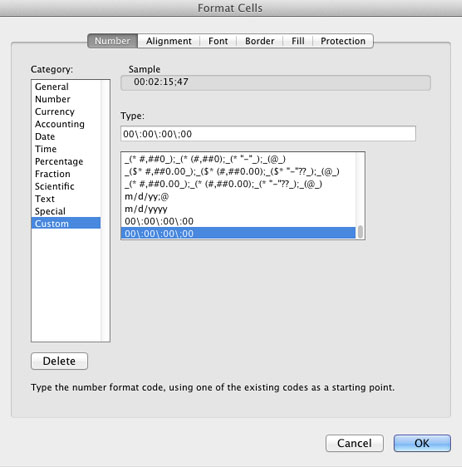This Excel formula grabs a timecode value from cell A1 and converts it into frames. The timecode should be in the format “01:01:01;01”. The formula uses 30 frames per second and does not calculate drop frames.

``````=VALUE(LEFT(A1,2))*60*60*30+VALUE(MID(A1,4,2))*60*30+VALUE(MID(A1,7,2))*30+RIGHT(A1,2)
``````

Example:
A1: 01:06:46;11
RESULT: 120191

This Excel formula grabs a number value from cell A2 and converts it into timecode.

``````=TEXT(MOD(INT(INT(INT(ABS(A2)/30)/60)/60),60),"00")&":"&TEXT(MOD(INT(INT(ABS(A2)/30)/60),60),"00")&":"&TEXT(MOD(INT(ABS(A2)/30),60),"00")&";"&TEXT(MOD(ABS(A2),30),"00")
``````

Example:
A2: 112019
RESULT: 01:02:13;29

You may want to replace “30” in that formula for a more accurate Frames Per Second value (e.g., 29.97 for NTSC, 25 for PAL).

This Excel formula calculates the difference between two timecode values and outputs “GOOD” if they are within 3 frames of each other. Otherwise, it outputs the actual difference as a timecode.

``````=IF((A4-A3)=MEDIAN((A4-A3),-3,3),"GOOD",TEXT(MOD(INT(INT(INT(ABS(A4-A3)/30)/60)/60),60),"00")&":"&TEXT(MOD(INT(INT(ABS(A4-A3)/30)/60),60),"00")&":"&TEXT(MOD(INT(ABS(A4-A3)/30),60),"00")&";"&TEXT(MOD(ABS(A4-A3),30),"00"))
``````

You may want to replace the value pair (-3,3) by a named cell containing a user determined error allowance. That way the formula will mark errors that fall withing the allowed interval as correct.

Example:
A3: 01:13:35;05
A4: 01:13:35;04
RESULT: GOOD

A3: 01:15:31;13
A4: 01:15:30;12
RESULT: 00:00:01;01

NOTE:
The formula “IF((A4-A3)=MEDIAN((A4-A3),-3,3)” calculates whether the value of A4-A3 falls within the values -3 and 3. If you were trying to determine whether these two values are 1 second apart, you would use -33 and 33 as your boundaries (30 frames equal 1 second).

This Excel formula will convert frames back into TimeCode. It will add 1 second to the final value, because Hulu requests that we give them the frame-accurate value of the middle of the commercial break.

``````=TEXT(MOD(INT(INT(INT(ABS(C3)/RFPS)/60)/60),60),"00")&":"&TEXT(MOD(INT(INT(ABS(C3)/RFPS)/60),60),"00")&":"&TEXT((MOD(INT(ABS(C3)/RFPS),60)+1),"00")&";"&TEXT(MOD(ABS(C3),RFPS),"00")
``````

This Excel formula will convert frames back into TimeCode. It will round up the seconds and zero out the frames, because YouTube only works with second-accurate commercial breaks. Since our commercial breaks last one second, we can guarantee we fall within the break by rounding up the seconds.

``````=TEXT(MOD(INT(INT(INT(ABS(C4)/RFPS)/60)/60),60),"00")&":"&TEXT(MOD(INT(INT(ABS(C4)/RFPS)/60),60),"00")&":"&TEXT((MOD(INT(ABS(C4)/RFPS),60)+1),"00")&";"&"00"
``````

RFPS in the formulas is a named cell containing the real FPS value.